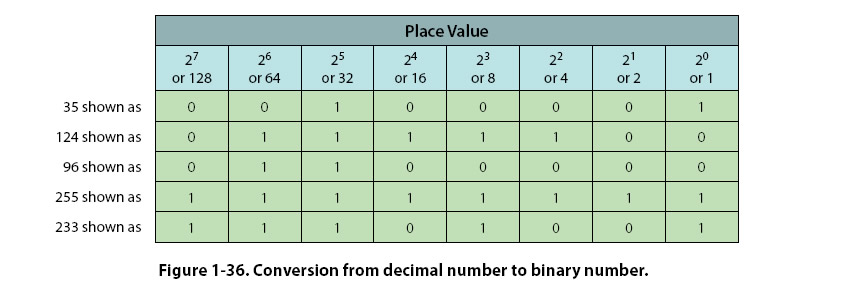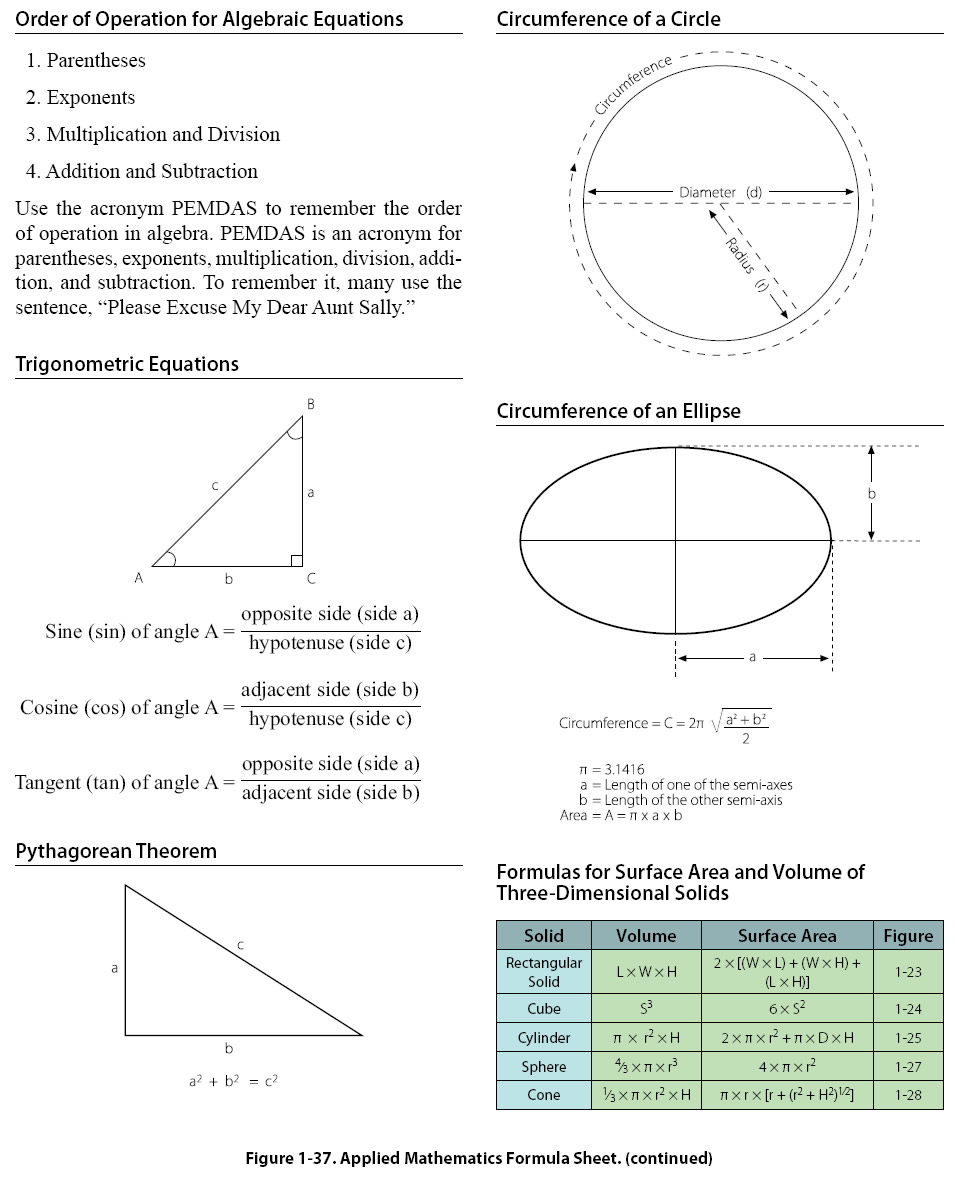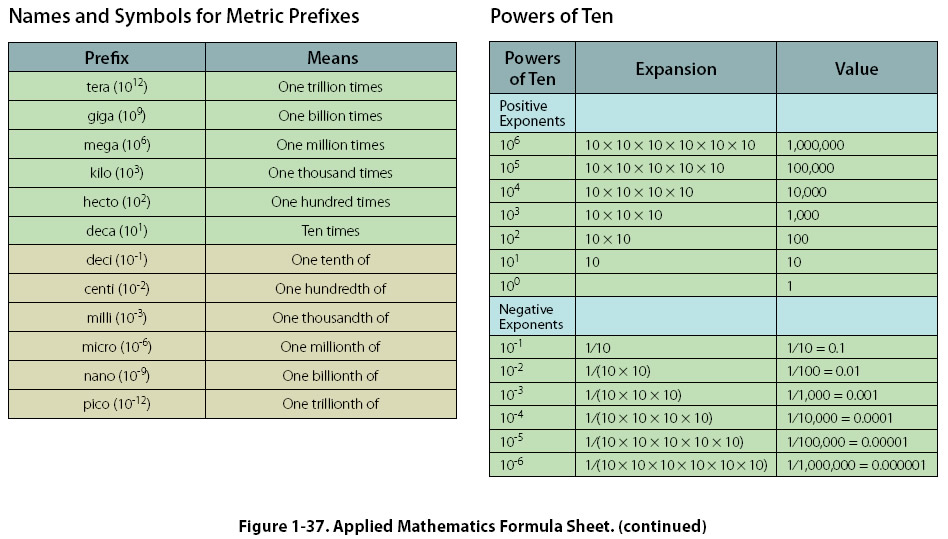Converting Decimal Numbers to Binary Numbers To convert a decimal number to a binary number, the place values in the binary system are used to create a sum of numbers that equal the value of the decimal number being converted. Start with the largest binary place value and subtract from the decimal number. Continue this process until all of the binary digits are determined. Example: Convert the decimal number 233 to a binary number. Start by subtracting 128 (the largest place value from the 8-bit binary number) from 233. 233 – 128 = 105 A “1” is placed in the first binary digit space: 1XXXXXXX. Continue the process of subtracting the binary number place values: 105 – 64 = 41 A “1” is placed in the second binary digit space: 11XXXXXX. 41 – 32 = 9 A “1” is placed in the third binary digit space: 111XXXXX. Since 9 is less than 16 (the next binary place value), a “0” is placed in the fourth binary digit space: 1110XXXX. 9 – 8 = 1 A “1” is placed in the fifth binary digit space: 11101XXX Since 1 is less than 4 (the next binary place value), a 0 is placed in the sixth binary digit space: 111010XX. Since 1 is less than 2 (the next binary place value), a 0 is placed in the seventh binary digit space: 1110100X. 1 – 1 = 0 A “1” is placed in the eighth binary digit space: 11101001. The decimal number 233 is equivalent to the binary number 11101001, as shown in Figure 1-36. Additional decimal number to binary number conversions are shown in Figure 1-36.©AvStop Online Magazine                                                                                                                                                      Contact Us              Return To Books